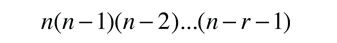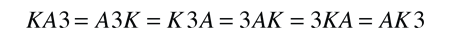# Permutations and Combinations

If you have a collection of n distinguishable objects, then the number of ways you can pick a number r of them (r < n) is given by the permutation relationship:Show

For example if you have six persons for tennis, then the number of pairings for singles tennis isBut this really double counts, because it treats the a:b match as distinct from the b:a match for players a and b. So in only 15 matches you could produce all distinguishable pairings. If you don't want to take into account the different permutations of the elements, then you must divide the above expression by the number of permutations of r which is r!. This result is called a "combination". The combination relationship is.

The number of tennis matches is then the combination.
Index

Statistics concepts

 HyperPhysics*****HyperMath R Nave
Go Back

# Permutations

The permutation relationship gives you the number of ways you can choose r objects or events out of a collection of n objects or events.As in all of basic probability, the relationships come from counting the number of ways specific things can happen, and comparing that number to the total number of possibilities. If you are making choices from n objects, then on your first pick you have n choices. On your second pick, you have n-1 choices, n-2 for your third choice and so forth. As illustrated before for 5 objects, the number of ways to pick from 5 objects is 5! .Note that your choice of 5 objects can take any order whatsoever, because your choice each time can be any of the remaining objects. So we say that there are 5 factorial = 5! = 5x4x3x2x1 = 120 ways to arrange five objects. In general we say that there are n! permutations of n objects.

Now if you are going to pick a subset r out of the total number of objects n, like drawing 5 cards from a deck of 52, then a counting process can tell you the number of different ways you can do that. For the first pick, you have n choices, then n-1 and so on down to n-r+1 for the last pick. The number of ways you can do it is thenwhich can be expressed in the standard formThe number of permutations of r objects out of n is sometimes what you need, but it has the drawback of overcounting if you are interested in the number of ways to get distinguishable collections of objects or events. For the purposes of card playing, the following ways of drawing 3 cards are equivalent:So the permutation relationship overcounts the number of ways to choose this combination if you don't want to make a distinction between them based on the order in which they were chosen. The factor of overcounting in this case is 6 = 3!, the number of permutations of 3 objects. The number of distinguishable collections of r objects chosen from n is obtained by dividing the permutation relationship by r! . It is usually writtenThis quantity nCr is called the "combination" and represents the probability of picking r distinguishable outcomes out of n without regard to the order of picking each outcome.

For a group of n objects or events which are broken up into k subsets, the above relationship is generalizable to give the number of distinguishable permutations of the n objects.Here the terms in the denominator are the populations of the k subsets. An application of this relationship is in determining the number of possible energy states in an energy distribution for distinguishable particles.

Index

Statistics concepts

 HyperPhysics*****HyperMath R Nave
Go Back